﻿ 双体船湍流脉动压力激励水下辐射噪声预报研究
 舰船科学技术2020, Vol. 42Issue (8): 38-42    DOI: 10.3404/j.issn.1672-7649.2020.08.007PDF

Research on prediction of noise radiation from turbulent pressure fluctuations on catamarans
GONG Cheng, LI Cong, ZHAO Chao, WANG Wei
Marine Design and Research Institute of China, Shanghai 200011, China
Abstract: By using large eddy simulation (LES), numerical computations of turbulent pressure fluctuations on a catamaran have been carried out both on scale model and full scale ship. Based on the result, a prediction of hydrodynamic noise radiating from catamarans has been executed by using acoustic FEM method. The simulation result matched well with the experiment, thus showed the effectiveness of LES method in simulating the distribution of turbulent pressure. Besides, based on the input of the turbulent pressure fluctuations, the radiation noise acquired by acoustic FEM method corresponded with the result of traditional SEA method in high-frequency stage, while in low-frequency stage, the result is higher than that of SEA method, which can be more reasonable to predict hydrodynamic noise of catamarans.
Key words: catamaran     turbulent pressure fluctuations     acoustic FEM     hydrodynamic noise
0 引　言

1 数值计算方法 1.1 大涡模拟控制方程

 $\phi = \overline \phi + \phi '{\text{，}}$ (1)

 $\frac{{\partial \rho }}{{\partial t}} + \frac{\partial }{{\partial {x_i}}}(\rho {\bar u_i}) = 0{\text{，}}$ (2)
 $\frac{\partial }{{\partial t}}(\rho {\bar u_i}) + \frac{\partial }{{\partial {x_j}}}(\rho {\bar u_i}{\bar u_j}) = \frac{\partial }{{\partial {x_j}}}(\mu \frac{{\partial {\sigma _{ij}}}}{{\partial {x_j}}}) - \frac{{\partial \bar p}}{{\partial {x_i}}} - \frac{{\partial {\tau _{ij}}}}{{\partial {x_j}}}{\text{，}}$ (3)

 $\frac{{\partial {{\bar u}_i}}}{{\partial {x_i}}} = 0{\text{，}}$ (4)
 $\frac{{\partial {{{\tilde {\bar u}}}_i}}}{{\partial t}} + \frac{\partial }{{\partial {x_j}}}({{\tilde {\bar u}}_i}{{\tilde {\bar u}}_j}) = - \frac{{\partial {\tilde {\bar p}}}}{{\partial {x_i}}} - \frac{{\partial {T_{ij}}}}{{\partial {x_j}}} + \frac{1}{{\rm{Re} }}\frac{{{\partial ^2}{\tilde {\bar u}}{}_i}}{{\partial {x_j}\partial {x_j}}}{\text{，}}$ (5)

 ${T_{ij}} = \widetilde {\overline {{u_i}{u_j}} } - {{\tilde {\bar u}}_i}{{\tilde {\bar u}}_j}{\text{。}}$ (6)
1.2 声学有限元法

 $p\left( {\vec r} \right) \approx \sum\limits_{i = 1}^n {{N_i}\left( {\vec r} \right){p_i}} = \left[ {{N_i}} \right]\left\{ {{p_i}} \right\}{\text{。}}$ (7)

 $\left\{ {\left[ {{K_a}} \right] + j\omega \left[ {{C_a}} \right] - {\omega ^2}\left[ {{M_a}} \right]} \right\}\left\{ {{p_i}} \right\} = \left\{ {{P_a}} \right\}{\text{，}}$ (8)

 $\left\{ {\left[ {{K_s}} \right] + j\omega \left[ {{C_s}} \right] - {\omega ^2}\left[ {{M_s}} \right]} \right\}\left\{ {{\delta _i}} \right\} = \left\{ {{F_s}} \right\}{\text{，}}$ (9)

 $\begin{split} & \left\{ {\left[ {\begin{array}{*{20}{c}} {{K_s}}&{{K_c}} \\ 0&{{K_a}} \end{array}} \right] + j\omega \left[ {\begin{array}{*{20}{c}} {{C_s}}&0 \\ 0&{{C_a}} \end{array}} \right] - {\omega ^2}\left[ {\begin{array}{*{20}{c}} {{M_s}}&0 \\ { - {\rho _0}K_c^{\rm{T}}}&{{M_a}} \end{array}} \right]} \right\}\\ &\left\{ {\begin{array}{*{20}{c}} {{\delta _i}} \\ {{p_i}} \end{array}} \right\} = \left\{ {\begin{array}{*{20}{c}} {{F_s}} \\ {{P_a}} \end{array}} \right\}{\text{。}}\end{split}$ (10)

2 湍流脉动压力的数值模拟 2.1 试验与数值模型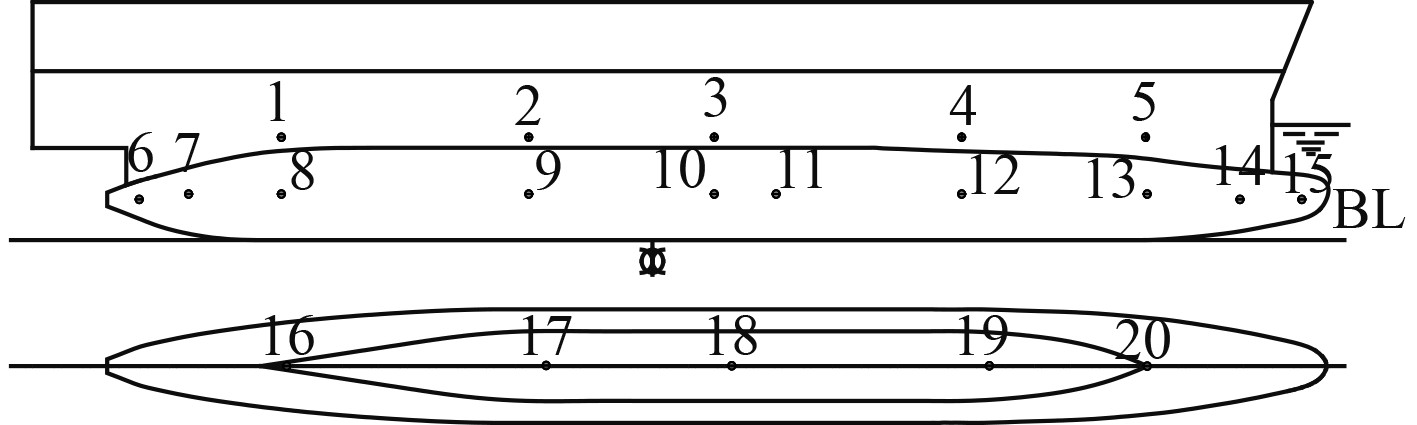图 1 测点布置 Fig. 1 Measuring-point arrangement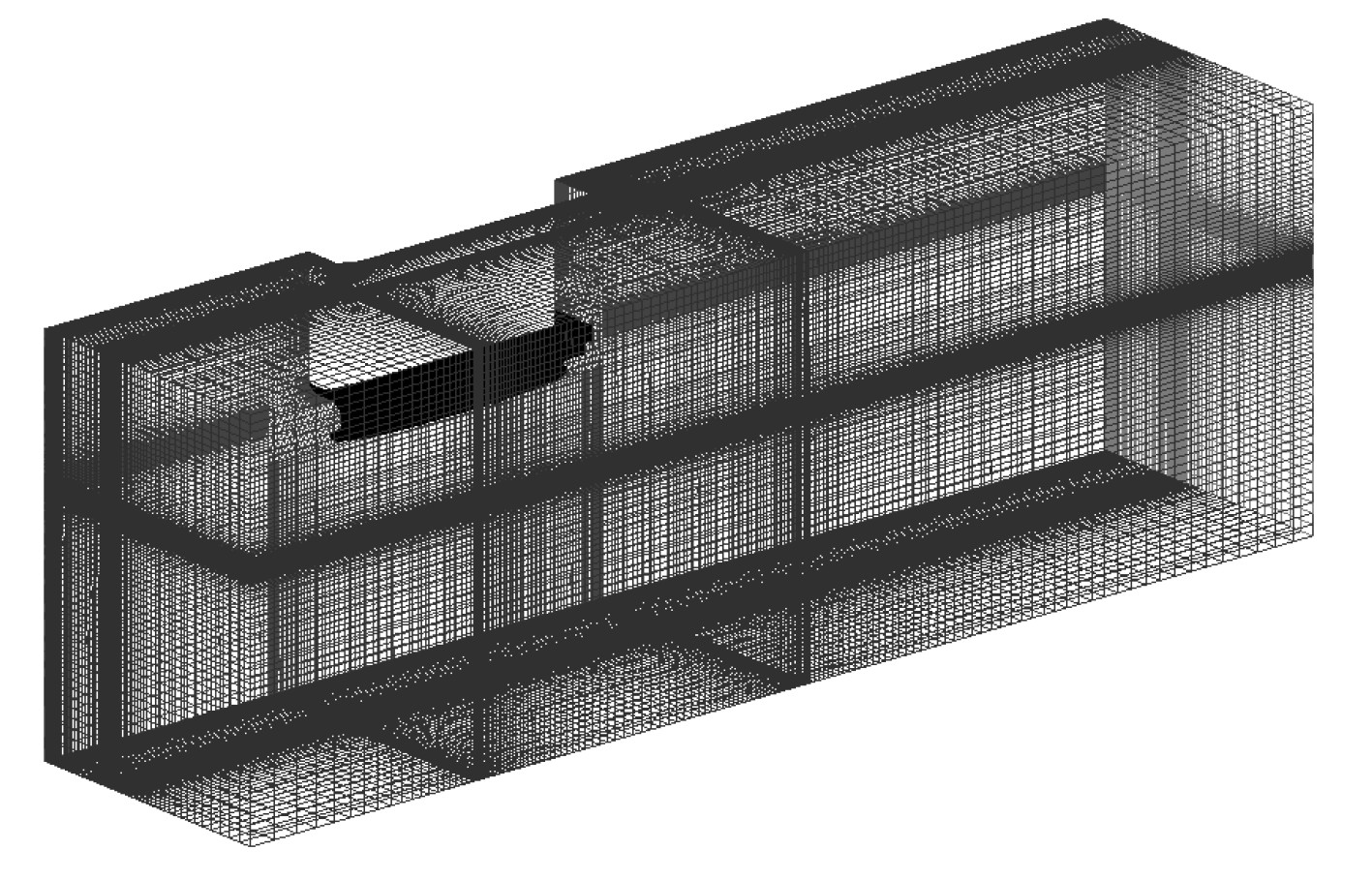图 2 双体船计算与网格划分 Fig. 2 Computational domain and meshing of the catamaran

2.2 数值计算结果 2.2.1 模型脉动压力计算与验证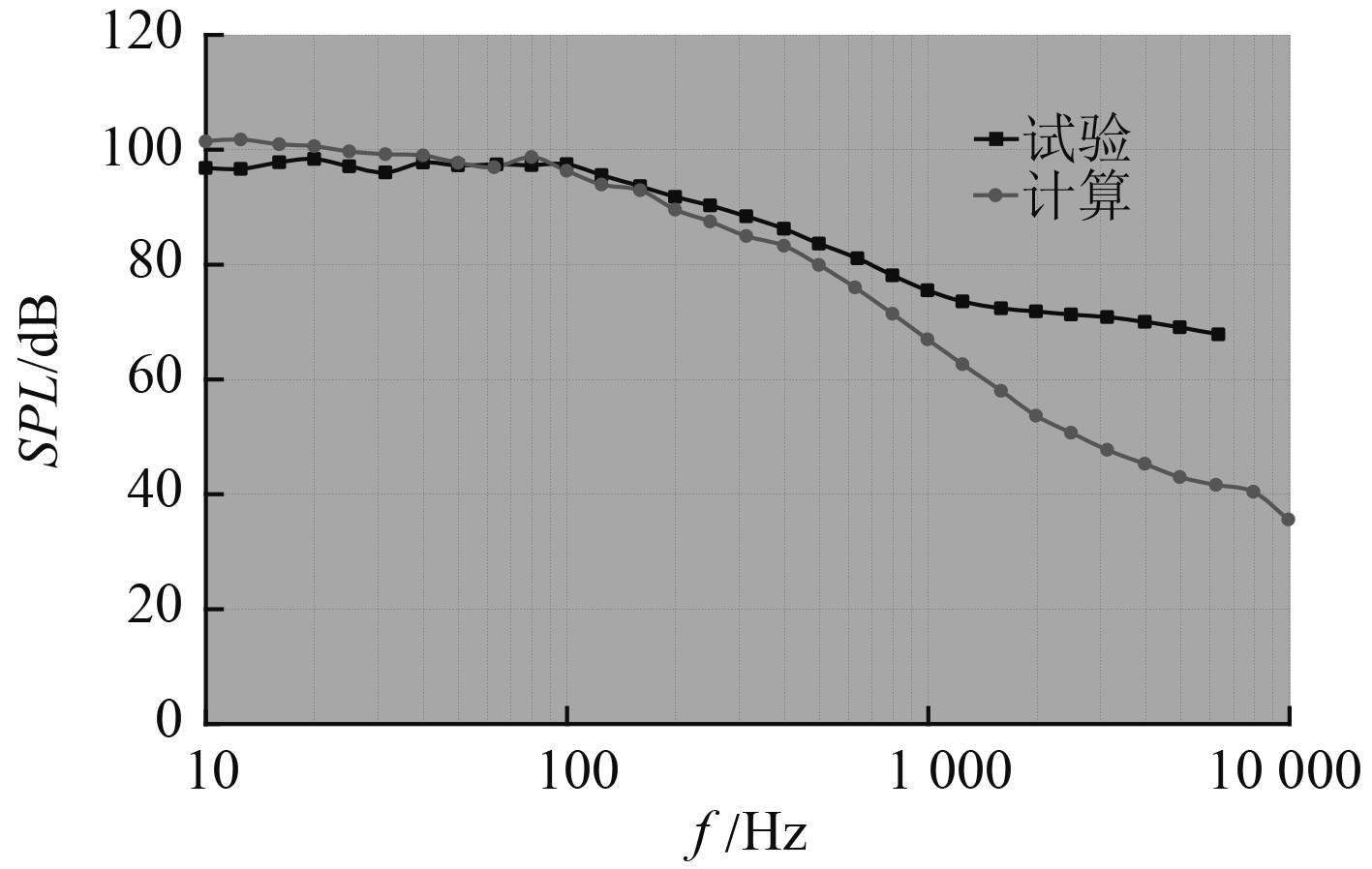图 3 P1点脉动压力计算与试验对比 Fig. 3 Comparison between simulation and experiment of turbulent pressure fluctuations at point P1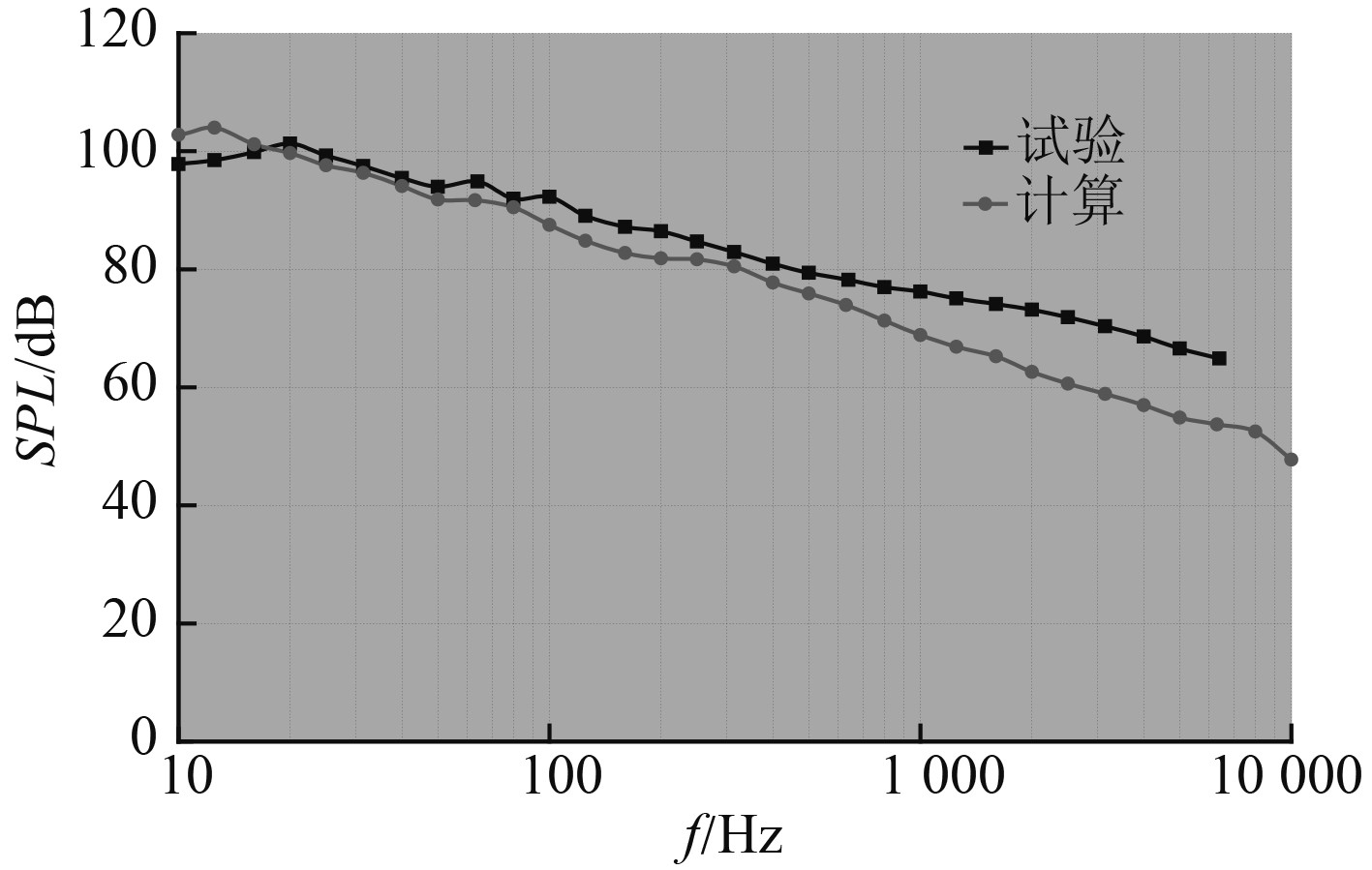图 4 P5点脉动压力计算与试验对比 Fig. 4 Comparison between simulation and experiment of turbulent pressure fluctuations at point P5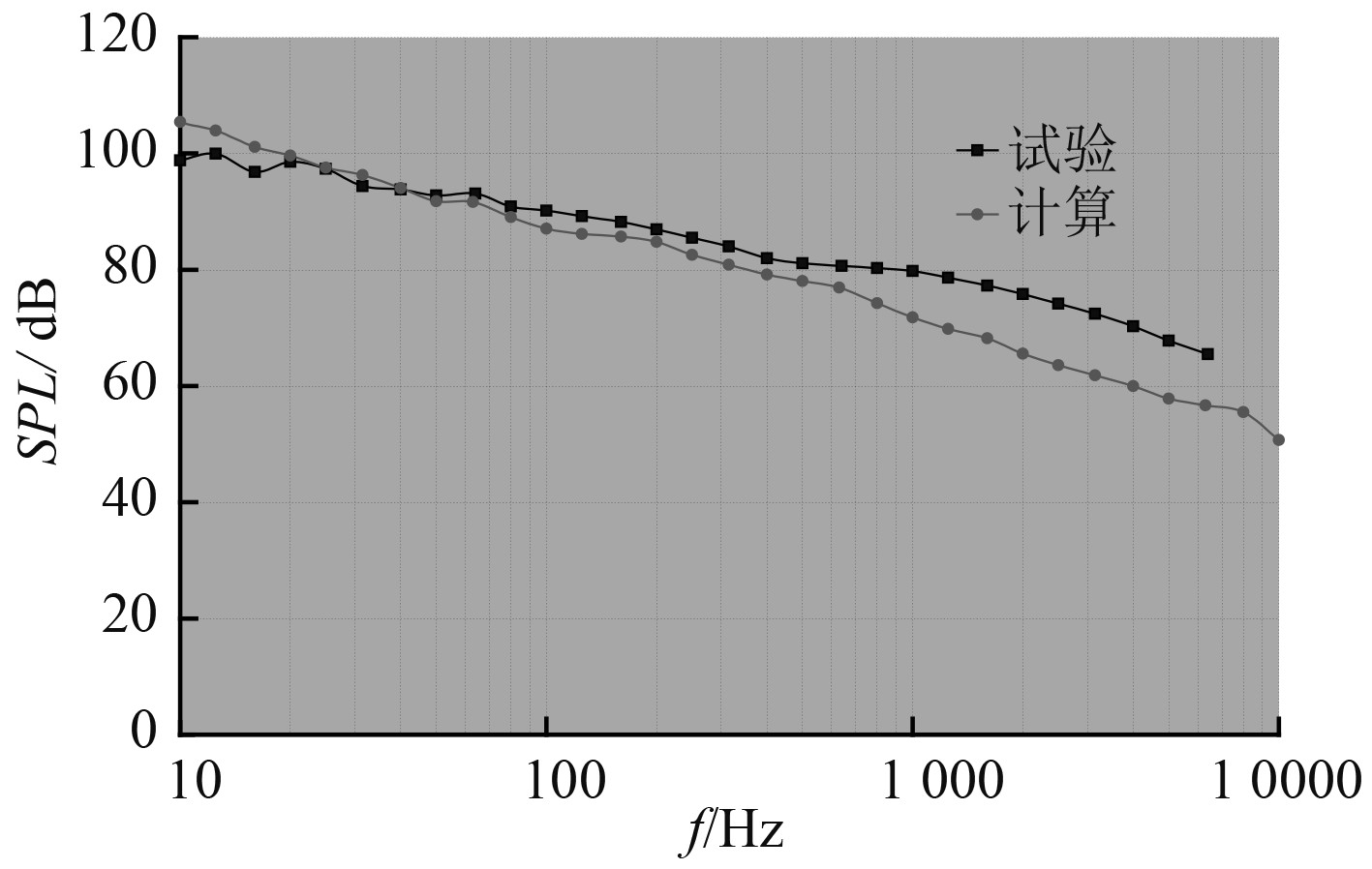图 5 P10点脉动压力计算与试验对比 Fig. 5 Comparison between simulation and experiment of turbulent pressure fluctuations at point P10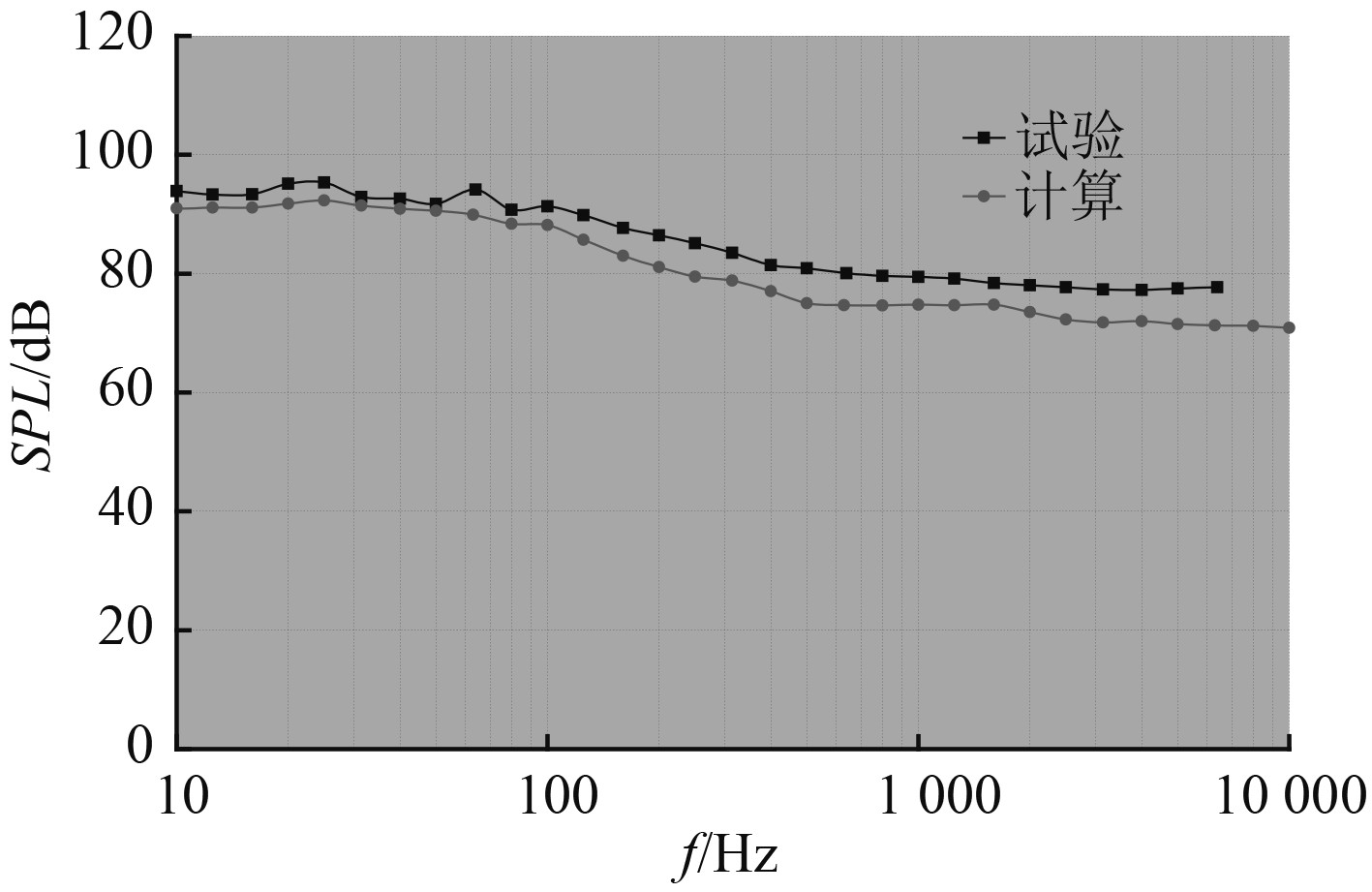图 6 P19点脉动压力计算与试验对比 Fig. 6 Comparison between simulation and experiment of turbulent pressure fluctuations at point P19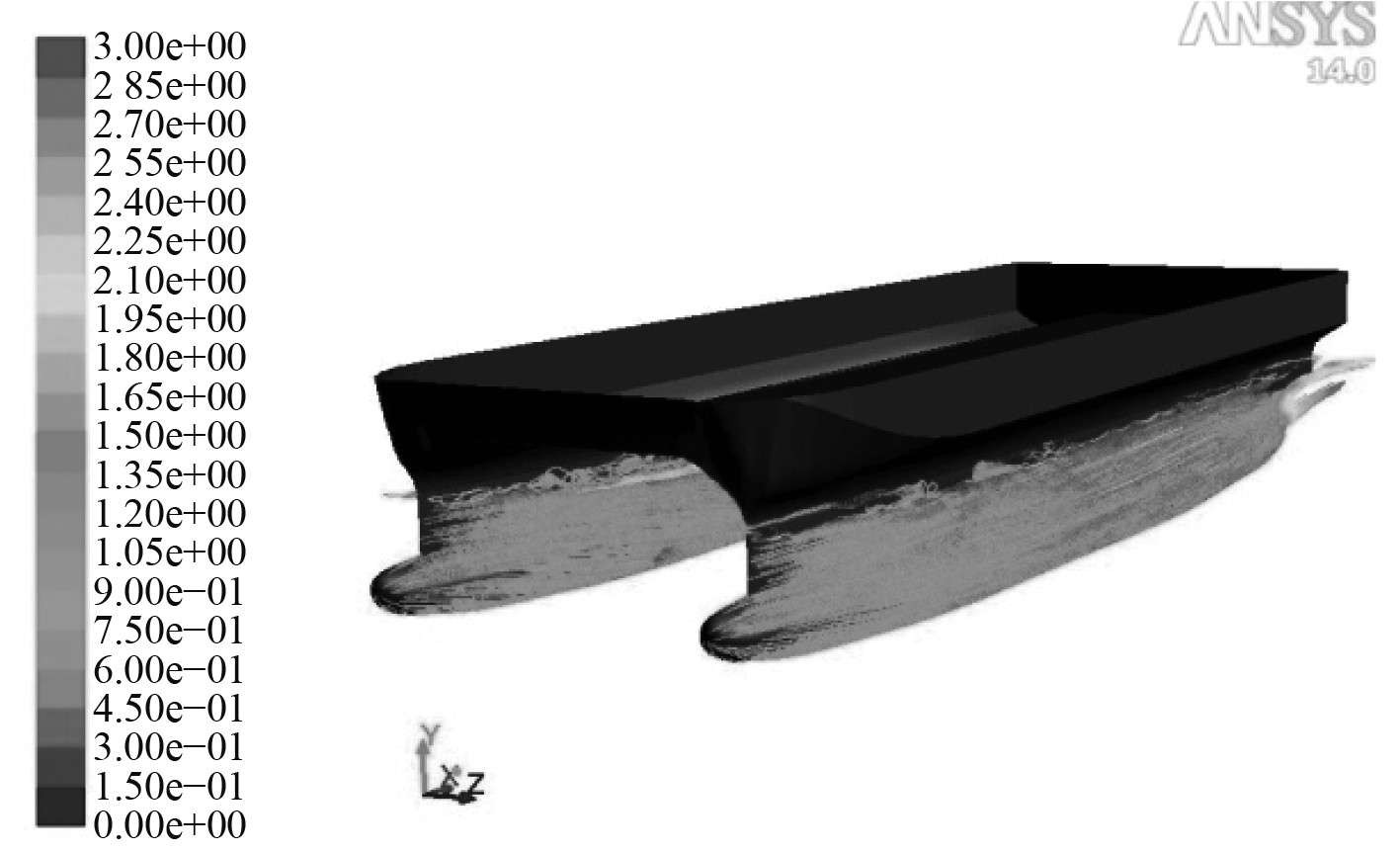图 7 实船表面流线分布 Fig. 7 Streamline distribution of full scale ship

2.2.2 实船脉动压力计算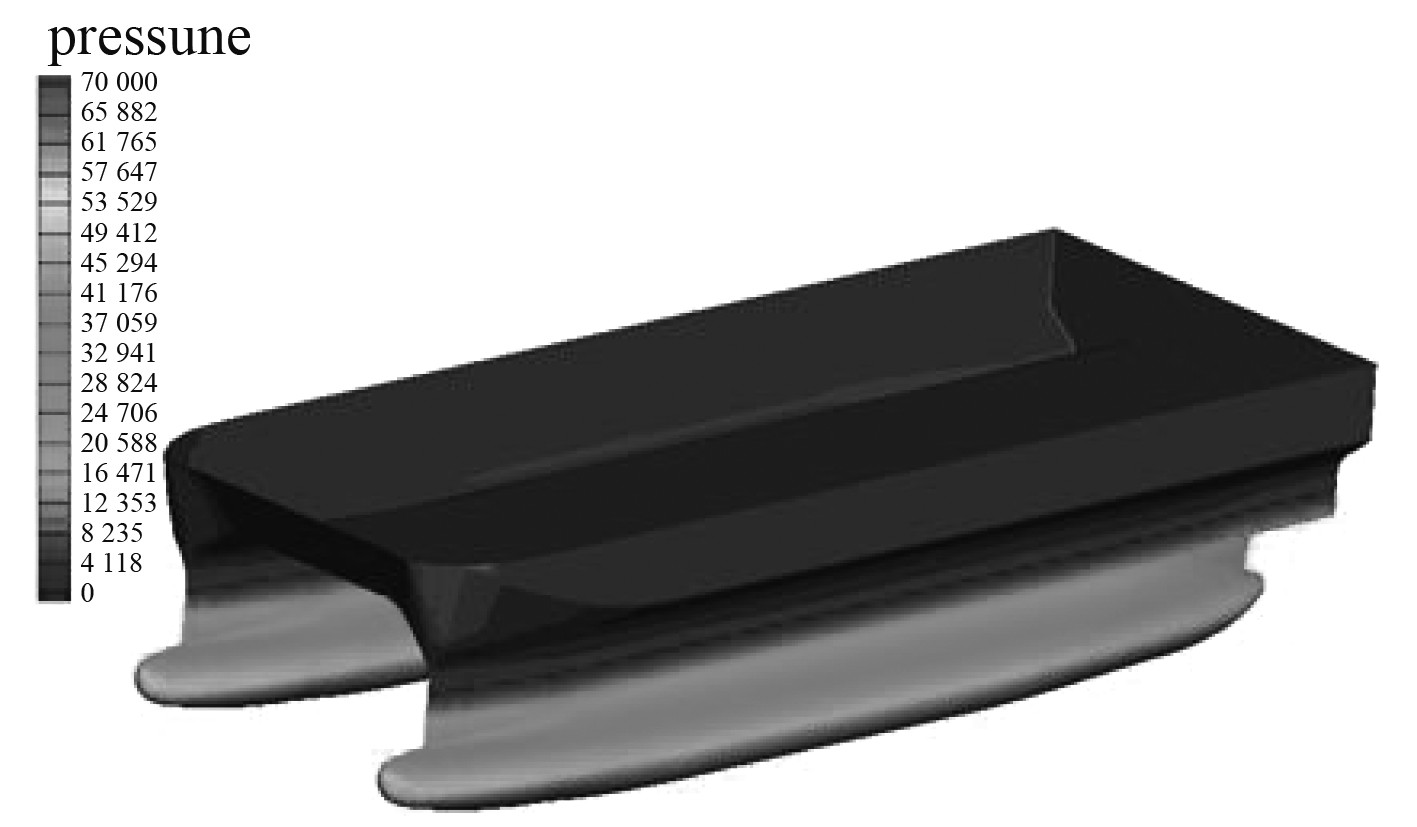图 8 实船水下部分压力分布 Fig. 8 Pressure distribution of underwater part of full scale ship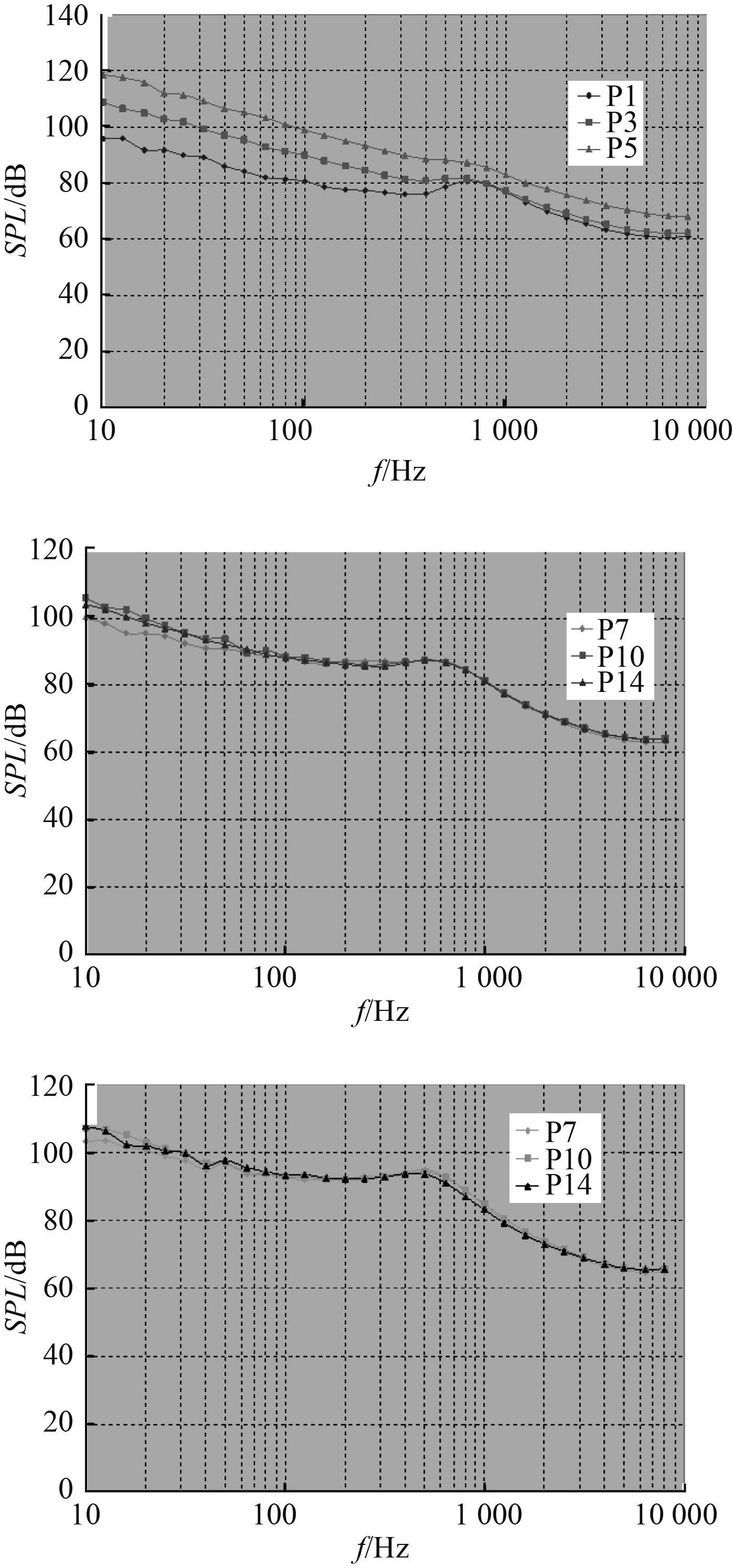图 9 实船各测点脉动压力（1/3 oct） Fig. 9 Turbulent pressure fluctuations of full scale ship at different points（1/3 oct）
3 船体水下辐射噪声预报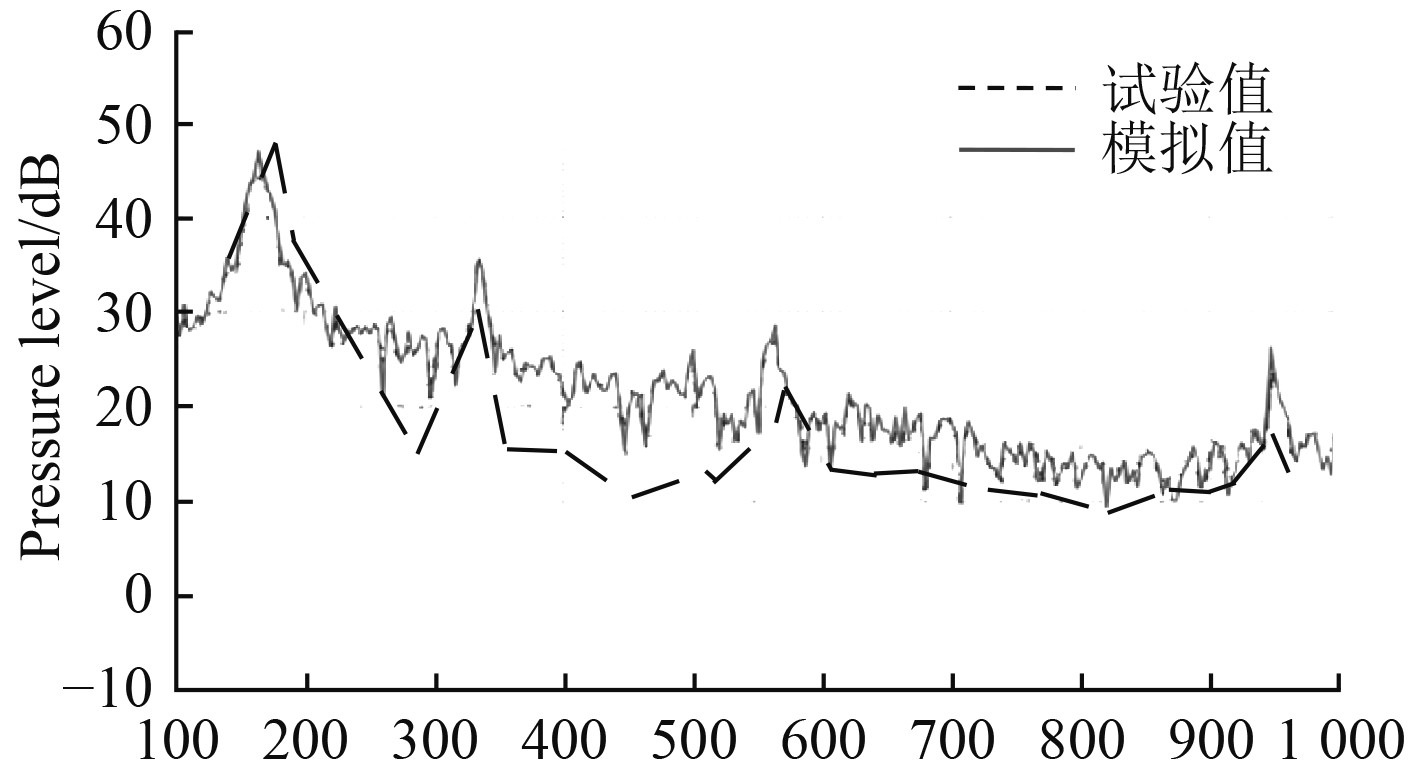图 10 试验及仿真计算结果 Fig. 10 Comparison between simulation and experiment result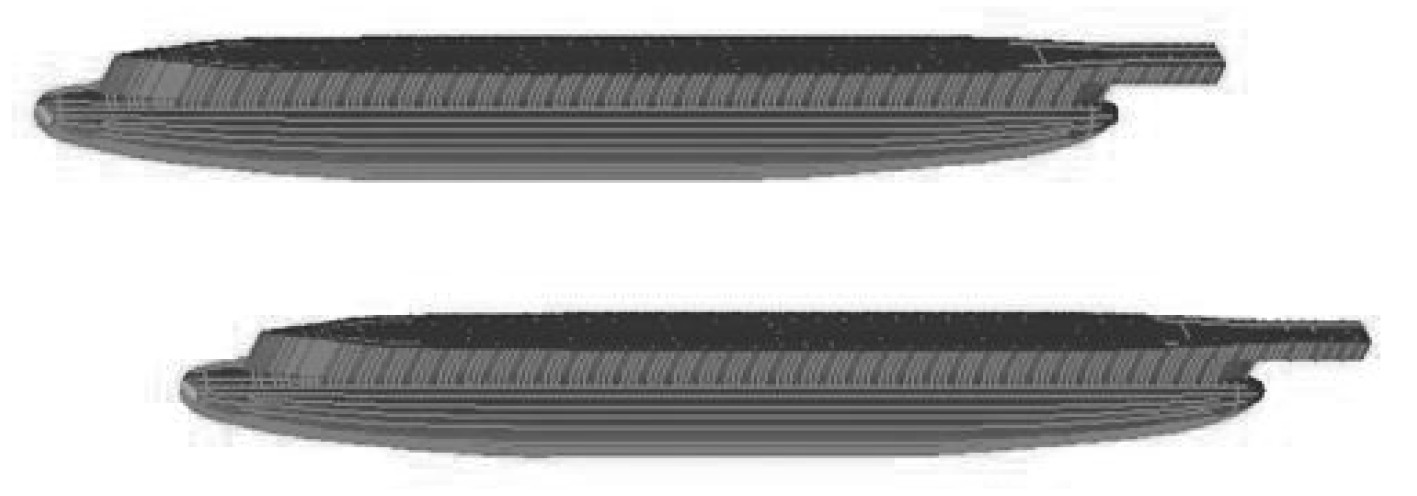图 11 双体船水下结构有限元模型 Fig. 11 FEM model of the catamaran’s underwater structure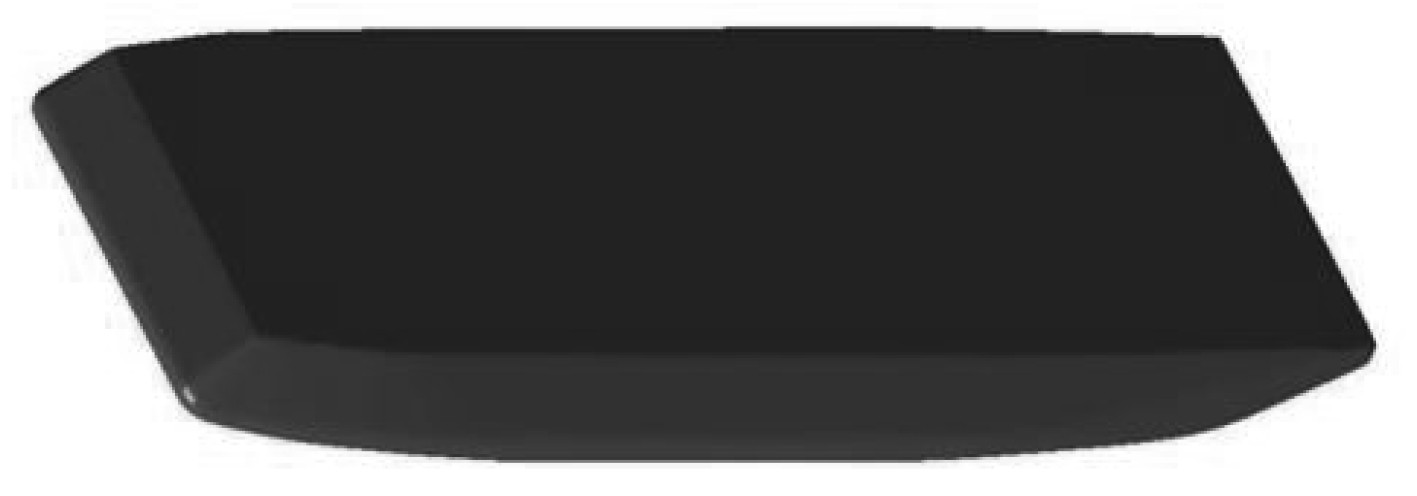图 12 外场流体区域有限元模型 Fig. 12 FEM model of fluid region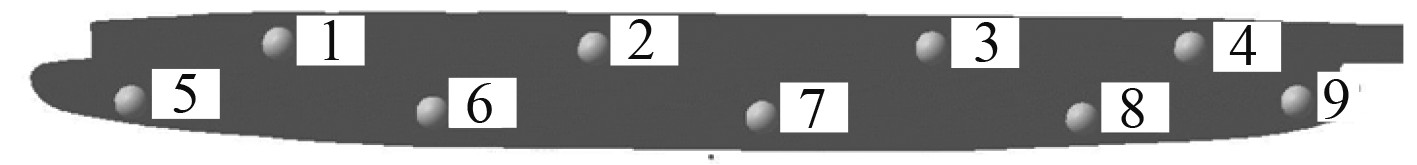图 13 振动测点位置 Fig. 13 Positions of vibration measuring-points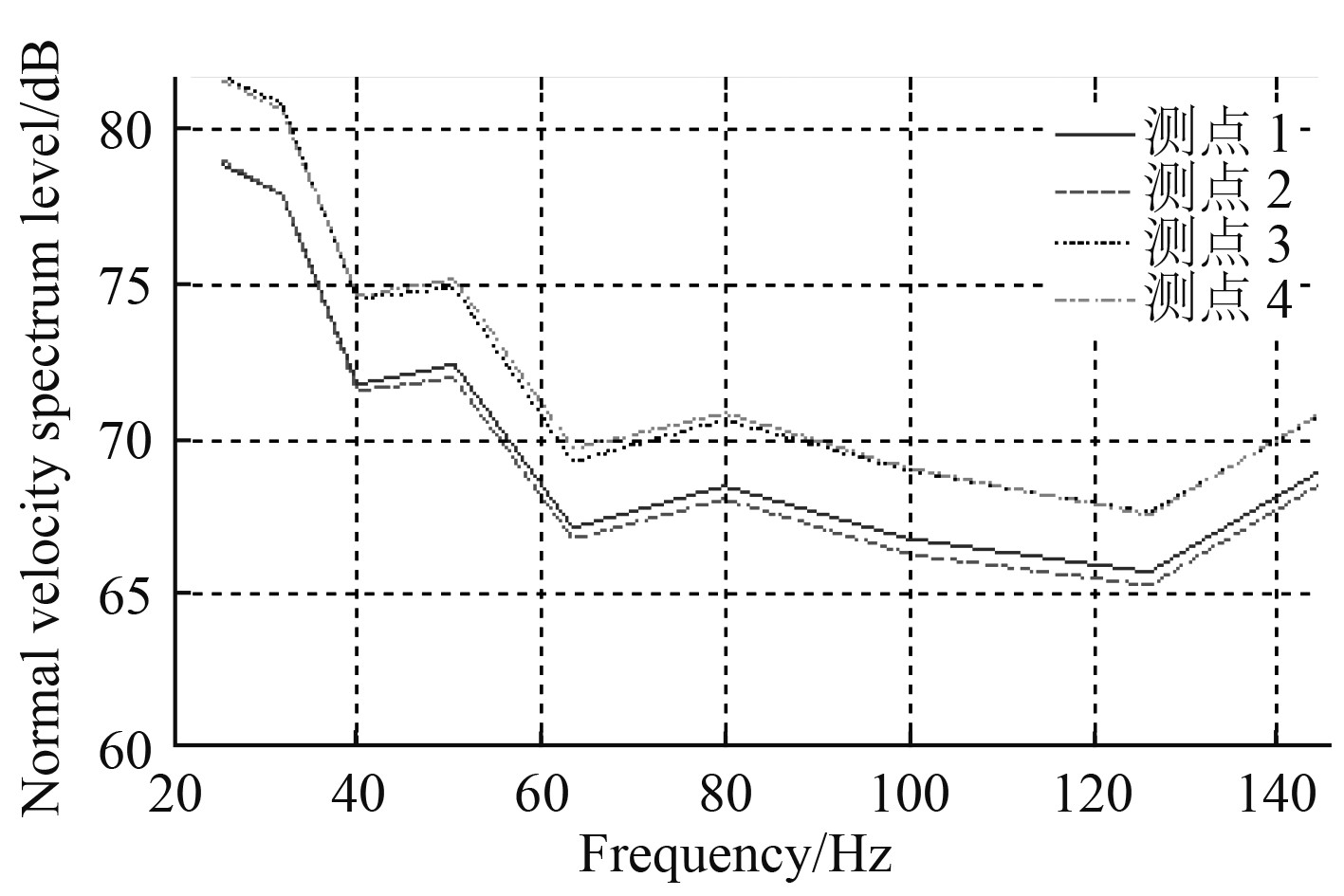图 14 支柱测点法向振速谱级（1/3 oct） Fig. 14 Normal vibration velocity spectrum level of measuring-points on pillar area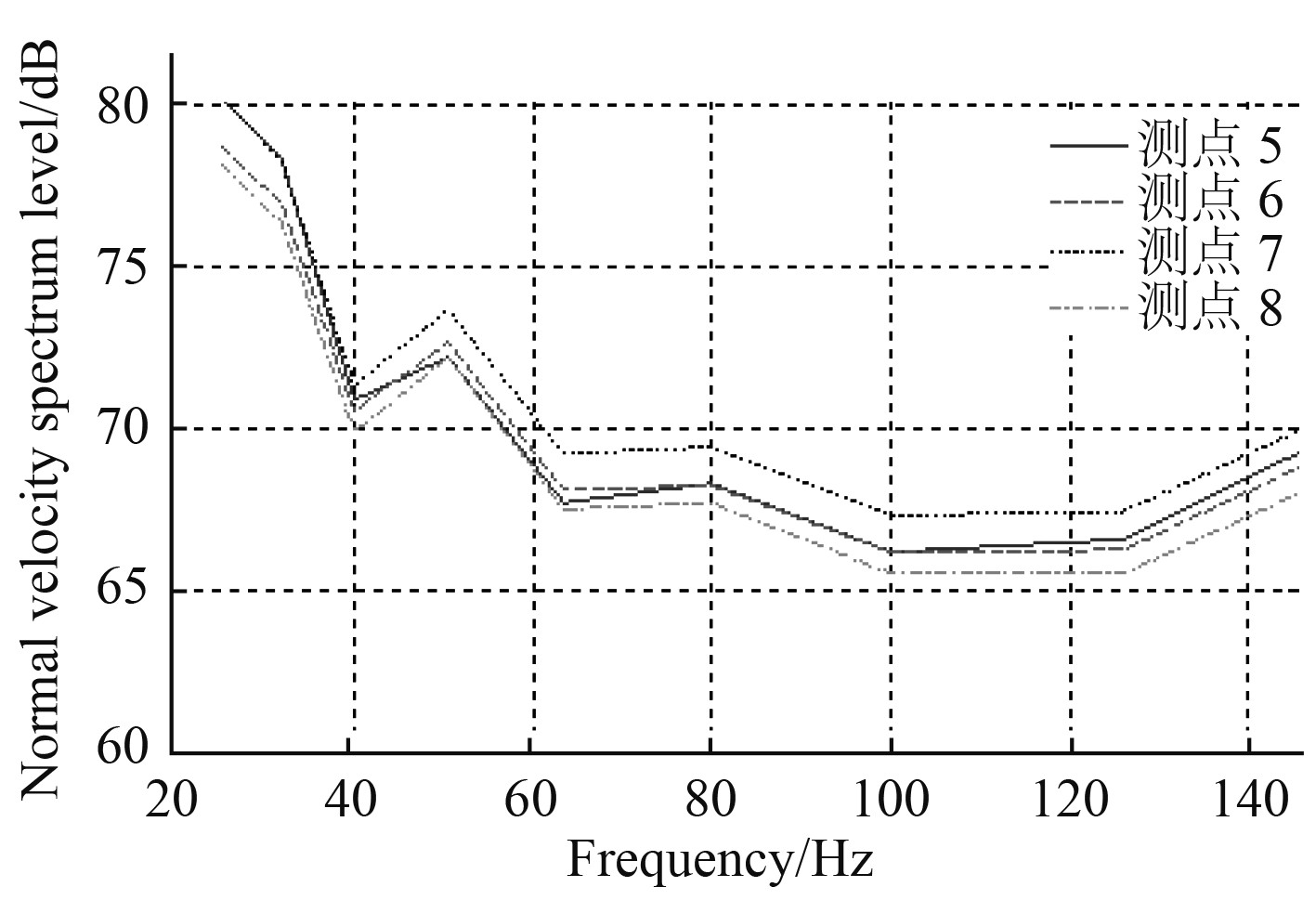图 15 潜体测点法向振速谱级（1/3 oct） Fig. 15 Normal vibration velocity spectrum level of measuring-points on submersible area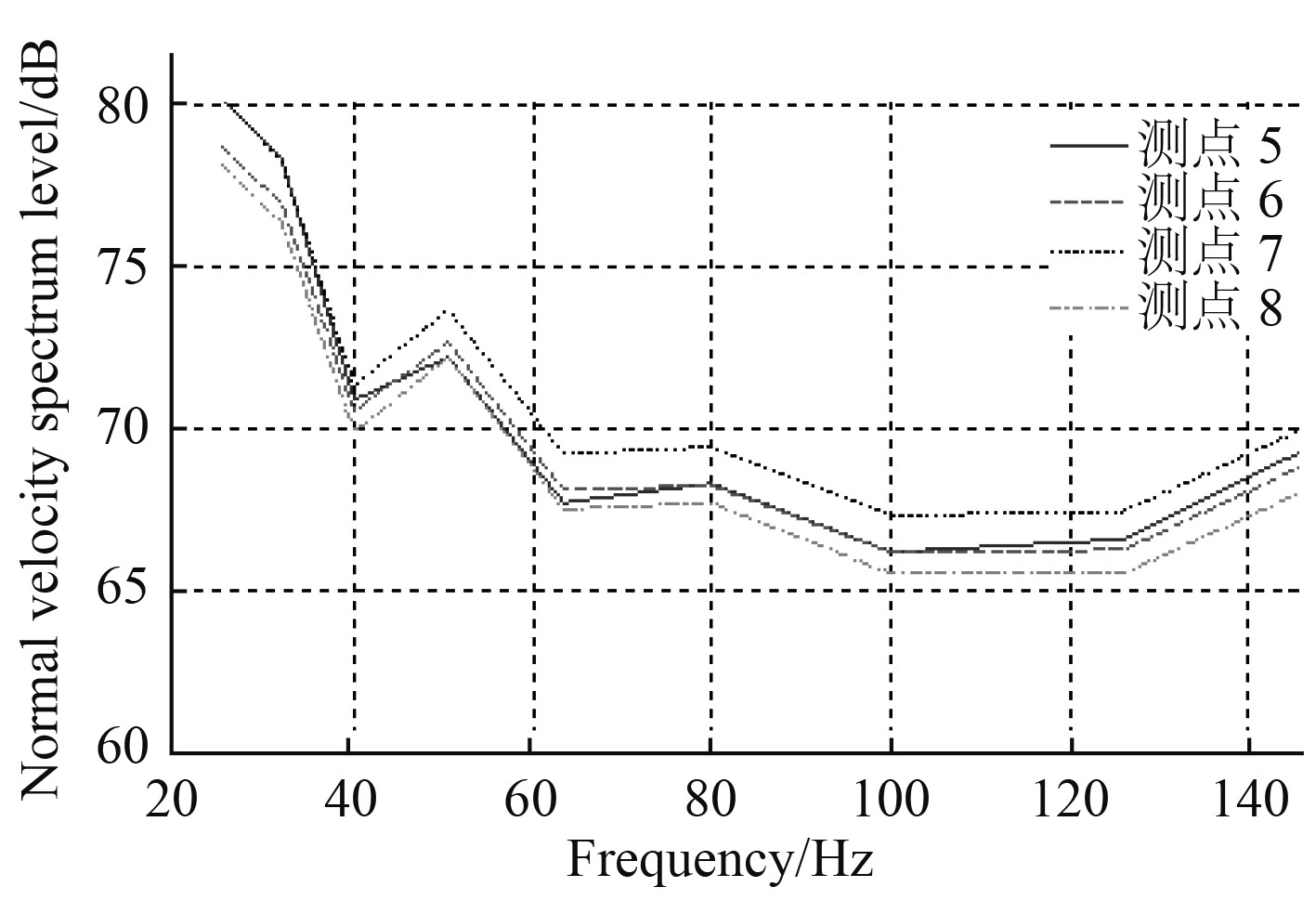图 16 有限元法与统计能量法计算结果对比 Fig. 16 Comparison between results from FEM and SEA method
4 结　语

  DAVIES H. G. Sound from turbulent boundary layer excited [J] panels , Journal. Acoust. Soc. Am., 1971;49(3): 878～889.  彭临慧, 陆建辉, 邱晓芳, 等. 水下回转体湍流边界层压力起伏谱经验公式[J]. 声学学报, 2001, 26(1): 51-54. PENG Lin-hui, LU Jian-hui, QIU Xiao-fang, et al. Empirical formula of turbulent boundary layer pressure fluctuation spectrum on underwater body of revolution[J]. ACTA ACUSTICA, Chinese version, 2001, 26(1): 51-54. DOI:10.3321/j.issn:0371-0025.2001.01.009  吕世金, 俞孟萨, 李东升. 水下航行体水动力辐射噪声预报方法研究[J]. 水动力学研究与进展, 2007, 22(4): 475-482. LV Shi-jin, YU Meng-sa, LI Dong-sheng. Prediction of hydrodynamic radiation noise of underwater vehicle[J]. Journal of hydrodynamics, 2007, 22(4): 475-482. DOI:10.3969/j.issn.1000-4874.2007.04.012  张楠, 沈泓萃, 姚惠之, 等. 水下航行体壁面脉动压力的大涡模拟研究[J]. 水动力学研究与进展, 2010, 25(1): 106-112. ZHANG Nan, SHEN Hong-cui, YAO Hui-zhi, et al. Large eddy simulation of wall pressure fluctuations of underwater vehicle[J]. Journal of Hydrodynamics, 2010, 25(1): 106-112. DOI:10.3969/j.issn.1000-4874.2010-01.015  张晓龙, 张楠, 吴宝山. 平板壁面湍流脉动压力及其波数—频率谱的大涡模拟计算分析研究[J]. 船舶力学, 2014, 18(10): 1151-1164. ZHANG Xiao-long, ZHANG Nan, WU Bao-shan. Computation of turbulent wall pressure fluctuation and its wavenumber -frequency spectrum using large eddy simulation[J]. Journal of Ship Mechanics, 2014, 18(10): 1151-1164. DOI:10.3969/j.issn.1007-7294.2014.10.001  江文成. 潜艇流噪声与流固耦合作用下流激噪声的数值模拟[D]. 上海: 上海交通大学, 2013. JIANG Wen-cheng. Numerical simulation on flow noise and flow-excited noise of submarine under fluid structure interaction[D]. Shanghai: Shanghai Jiao Tong University, 2013.  BRUN C, et al. (Eds.) Numerical Simulation of turbulent flows & noise generation [U]. Springer-Verlag Berlin Heidelberg. 2009.  HEATWOLE CM, FRANCHEK MA, BERNHARD RJ. Robust feedback control of flow induced structural radiation of sound [V]. Herrick Laboratory Internal Report, #0278-1, 7-97.  HEATWOLE CM, FRANCHEK MA, BERNHARD RJ. A robust feedback controller implementation for flow induced structural radiation of sound [J]. In: Noise-Con 96, Seattle, Wash, 1996, 62-357.